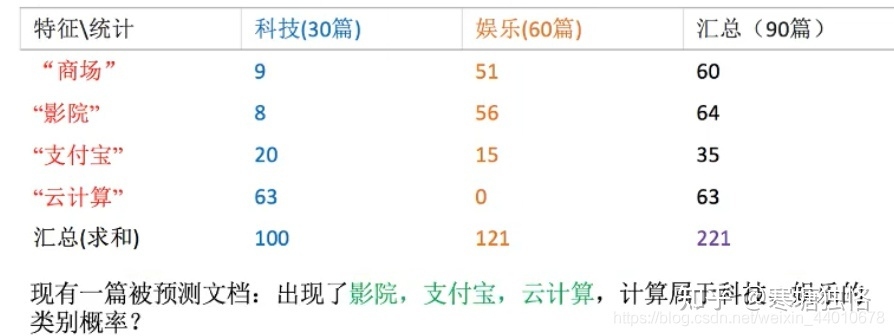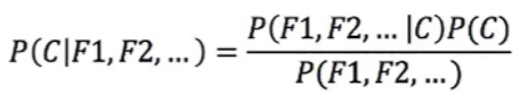# 例子• C为文档的类别（财经，体育，军事……）
• F1，F2为文档中的关键词

``````P(科技|影院，支付宝，云计算)=
P(影院，支付宝，云计算|科技)*P(科技)=
(8/100)(20/100)(63/100)(30/90)=0.005
``````
``````P(娱乐|影院，支付宝，云计算)=
P(影院，支付宝，云计算|娱乐)*P(娱乐)=
(56/121)(15/121)(0/121)(60/90)=0
``````

## 解决问题：拉普拉斯平滑系数• `a`：是指定的系数，一般为1
• `m`：是训练文档中统计出的特征词个数
• `Ni`：原来 `P(F1|C)` 中的分子
• `N`：原来 `P(F1|C)` 中的分母

``````P(科技|影院，支付宝，云计算)=
P(影院，支付宝，云计算|科技)*P(科技)=
((8+1)/(100+1*4))((1+20)/(100+1*4))((1+63)/(100+1*4))(30/90)
``````
``````P(娱乐|影院，支付宝，云计算)=
P(影院，支付宝，云计算|娱乐)*P(娱乐)=
((1+56)/(121+1*4))((1+15)/(121+1*4))((1+0)/(121+1*4))(60/90)
``````

# API 声明

``````class sklearn.naive_bayes.MultinomialNB(
alpha=1.0,
fit_prior=True,
class_prior=None
)
``````

• alpha:拉普拉斯平滑系数，默认为1.0。注意拉普拉斯平滑系数不是超参数，并不会影响最后的结果。
• fit_prior : 布尔值, 可不填 (默认为True)
是否学习先验概率 `P(Y=c)`。如果设置为false，则不使用先验概率，而使用统一先验概率（uniform prior），即认为每个标签类出现的概率是 `1 / n_classes`
• class_prior：形似数组的结构，结构为(n_classes, )，可不不填（默认为None）
类的先验概率P(Y=c)。如果没有给出具体的先验概率则自动根据数据来进行计算。

# 使用例子

### 训练模型、预测

``````import numpy as np
from sklearn.naive_bayes import MultinomialNB

# 测试数据
X = np.array([[2,1,1,3,3],[2,0,2,1,1],[0,2,1,0,4],[1,0,3,1,3],[1,3,3,3,2]])
# 测试标签
y = np.array([1, 2, 3, 4, 5])
clf = MultinomialNB()

# 训练模型
clf.fit(X, y)

# 预测
print(clf.predict(X[2:3]))
``````

``````
``````

### class_prior 参数

``````import numpy as np
from sklearn.naive_bayes import MultinomialNB
X = np.array([[2,1,1,3,3],[2,0,2,1,1],[0,2,1,0,4],[1,0,3,1,3],[1,3,3,3,2]])
y = np.array([1, 2, 3, 4, 5])
clf = MultinomialNB()
clf.fit(X, y)
## 观察各类标记的平滑先验概率对数值
print(clf.class_log_prior_)
## 指定先验概率
clf1 = MultinomialNB(alpha=1.0,fit_prior=False,class_prior=[0.3,0.2,0.1,0.2,0.2])
clf1.fit(X,y)
## 观察各类标记的平滑先验概率对数值
print(clf1.class_log_prior_)
``````

``````[-1.60943791 -1.60943791 -1.60943791 -1.60943791 -1.60943791]
[-1.2039728  -1.60943791 -2.30258509 -1.60943791 -1.60943791]
``````

### fit_prior 参数为 False

`fit_prior` 参数为 `False``class_prior=None`，则各类标记的先验概率相同等于类标记总个数N分之一

``````import numpy as np
from sklearn.naive_bayes import MultinomialNB
X = np.array([[2,1,1,3,3],[2,0,2,1,1],[0,2,1,0,4],[1,0,3,1,3],[1,3,3,3,2]])
y = np.array([1, 2, 3, 4, 5])
clf = MultinomialNB(fit_prior=False,class_prior=None)
clf.fit(X, y)
## 观察各类标记的平滑先验概率对数值
print(clf.class_log_prior_)

# 验证是否是1/5
print(np.log(0.2))
``````

``````[-1.60943791 -1.60943791 -1.60943791 -1.60943791 -1.60943791]
-1.6094379124341003
``````

### fit_prior参数为True

`fit_prior` 参数为 `True``class_prior=None`，则各类标记的先验概率等于各类标记个数除以各类标记个数之和（将y的类别稍加改动即可）

``````import numpy as np
from sklearn.naive_bayes import MultinomialNB
X = np.array([[2,1,1,3,3],[2,0,2,1,1],[0,2,1,0,4],[1,0,3,1,3],[1,3,3,3,2]])
y = np.array([1, 1, 2, 3, 4])
clf = MultinomialNB(fit_prior=True,class_prior=None)
clf.fit(X, y)
## 观察各类标记的平滑先验概率对数值
print(clf.class_log_prior_)

# 验证是否是2/5 、 1/5 、1/5 、1/5
print(np.log(2/5),np.log(1/5),np.log(1/5),np.log(1/5))
``````

``````[-0.91629073 -1.60943791 -1.60943791 -1.60943791]
-0.916290731874155 -1.6094379124341003 -1.6094379124341003 -1.6094379124341003
``````